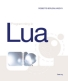This first edition was written for Lua 5.0. While still largely relevant for later versions, there are some differences.
The fourth edition targets Lua 5.3 and is available at Amazon and other bookstores.
By buying the book, you also help to support the Lua project.

## 28.3 – Object-Oriented Access

Our next step is to transform our new type into an object, so that we can operate on its instances using the usual object-oriented syntax, such as

```    a = array.new(1000)
print(a:size())     --> 1000
a:set(10, 3.4)
print(a:get(10))    --> 3.4
```

Remember that `a:size()` is equivalent to `a.size(a)`. Therefore, we have to arrange for the expression `a.size` to return our `getsize` function. The key mechanism here is the `__index` metamethod. For tables, this metamethod is called whenever Lua cannot find a value for a given key. For userdata, it is called in every access, because userdata have no keys at all.

Assume that we run the following code:

```    local metaarray = getmetatable(array.new(1))
metaarray.__index = metaarray
metaarray.set = array.set
metaarray.get = array.get
metaarray.size = array.size
```
In the first line, we create an array only to get its metatable, which we assign to `metaarray`. (We cannot set the metatable of a userdata from Lua, but we can get its metatable without restrictions.) Then we set `metaarray.__index` to `metaarray`. When we evaluate `a.size`, Lua cannot find the key `"size"` in object `a`, because the object is a userdatum. Therefore, Lua will try to get this value from the field `__index` of the metatable of `a`, which happens to be `metaarray` itself. But `metaarray.size` is `array.size`, so `a.size(a)` results in `array.size(a)`, as we wanted.

Of course, we can write the same thing in C. We can do even better: Now that arrays are objects, with their own operations, we do not need to have those operations in the table `array` anymore. The only function that our library still has to export is `new`, to create new arrays. All other operations come only as methods. The C code can register them directly as such.

The operations `getsize`, `getarray`, and `setarray` do not change from our previous approach. What will change is how we register them. That is, we have to change the function that opens the library. First, we need two separate function lists, one for regular functions and one for methods:

```    static const struct luaL_reg arraylib_f [] = {
{"new", newarray},
{NULL, NULL}
};

static const struct luaL_reg arraylib_m [] = {
{"set", setarray},
{"get", getarray},
{"size", getsize},
{NULL, NULL}
};
```
The new version of `luaopen_array`, the function that opens the library, has to create the metatable, to assign it to its own `__index` field, to register all methods there, and to create and fill the `array` table:
```    int luaopen_array (lua_State *L) {
luaL_newmetatable(L, "LuaBook.array");

lua_pushstring(L, "__index");
lua_pushvalue(L, -2);  /* pushes the metatable */
lua_settable(L, -3);  /* metatable.__index = metatable */

luaL_openlib(L, NULL, arraylib_m, 0);

luaL_openlib(L, "array", arraylib_f, 0);
return 1;
}
```
Here we use another feature from `luaL_openlib`. In the first call, when we pass `NULL` as the library name, `luaL_openlib` does not create any table to pack the functions; instead, it assumes that the package table is on the stack, below any occasional upvalues. In this example, the package table is the metatable itself, which is where `luaL_openlib` will put the methods. The next call to `luaL_openlib` works regularly: It creates a new table with the given name (`array`) and registers the given functions there (only `new`, in this case).

As a final touch, we will add a `__tostring` method to our new type, so that `print(a)` prints `array` plus the size of the array inside parentheses (for instance, `array(1000)`). The function itself is here:

```    int array2string (lua_State *L) {
NumArray *a = checkarray(L);
lua_pushfstring(L, "array(%d)", a->size);
return 1;
}
```
The `lua_pushfstring` function formats the string and leaves it on the stack top. We also have to add `array2string` to the list `arraylib_m`, to include it in the metatable of array objects:
```    static const struct luaL_reg arraylib_m [] = {
{"__tostring", array2string},
{"set", setarray},
...
};
```